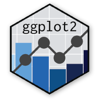Show Sidebar Hide Sidebar# aes in ggplot2

How assign aesthetics in ggplot2 and R.

### New to Plotly?

Plotly's R library is free and open source!
You can set up Plotly to work in online or offline mode.
We also have a quick-reference cheatsheet (new!) to help you get started!

### Version Check

Version 4 of Plotly's R package is now available!
Check out this post for more information on breaking changes and new features available in this version.

library(plotly)
packageVersion('plotly')

##  '4.9.1'


### Fill

library(plotly)

ds <- data.frame(x = 1:10,
y = sample(10:30, size = 10),
group = LETTERS[1:2])

# Use the fill aesthetic to specify the fill color for the bars
p <- ggplot(ds, aes(x, y)) +
geom_bar(aes(fill = group), stat = "identity") +
ggtitle("Filled bar chart")

p <- ggplotly(p)

p


### Group

library(plotly)

x1 <- 1:100
x2 <- x1 + 100
x3 <- x2 + 100

x <-  c(x1, x2, x3)
y <- c(2*x1, 5*x2, -2*x3)

group <- c(rep("A", length(x1)),
rep("B", length(x2)),
rep("C", length(x3)))

ds <- data.frame(x, y, group)

# Use the group aesthetic to ensure lines are drawn separately for each group
p <- ggplot(ds, aes(x, y)) +
geom_line(aes(group = group, color = group), size = 2) +
ggtitle("Group specific line chart")

p <- ggplotly(p)

p


### Label

library(plotly)

ds <- data.frame(x = rnorm(10),
y = rnorm(10),
group = LETTERS[1:2])

p <- ggplot(ds, aes(x, y)) +
geom_point(aes(color = group), size = 7) +
geom_text(aes(label = group), size = 4) +
ggtitle("Annotation with labels")

p <- ggplotly(p)

p


### Shape

library(plotly)

ds <- data.frame(x = letters[1:5],
y = rnorm(20),
group = LETTERS[1:4])

# Use aes shape to map individual points and or different groups to different shapes
p <- ggplot(ds, aes(x, y)) +
geom_point(aes(color = group, shape = group), size = 5) +
geom_line(aes(group = group, linetype = group)) +
ggtitle("Groupwise shapes and line types")

p <- ggplotly(p)

p


Inspired by ggplot2 documentation